SYLLABUS  Previous: 4.5 Methods for European  Up: 4.5 Methods for European  Next: 4.5.2 Expected value of

4.5.1 Forecast possible realizations of the underlying asset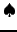[ SLIDE scheme - initialization - code - run || VIDEO modem - LAN - DSL]

From the definition of stochastic increments in sect.3.3.1, it is easy to propose an approximation for the uncertain evolution of the underlying asset price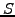, using a finite number of steps in time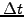, which result in correspondingly small increments in the price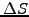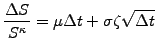(4.5.1#eq.1)

As previously,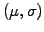are the drift and the volatility measured from the market,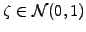is a normally distributed random number generated anew for each step and the parameter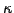is chosen to reproduce a normal walk with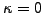or a log-normal walk with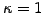.
Starting from (k=0,...,numberOfRealisations-1) samples modeling each one possible evolution of the asset (currentState[k]) and an equal number of markers (mark[k]) to account for barriers in exotic options, the initialization in SamplingSolution.java has been implemented as
numberOfRealisations = runData.getParamValueInt("Walkers");
strike  = runData.getParamValue("StrikePrice");
kappa   = runData.getParamValue("LogNkappa");
//Separable
if( (Math.abs(kappa)<0.001) ||(Math.abs(kappa-1.)<0.001)){
currentState = new double[numberOfRealisations];
for (k=0; k<numberOfRealisations; k++) currentState[k]=strike;
//Barriers
if(scheme.equals(vmarket.MCIN)||scheme.equals(vmarket.MCINPP)){
mark = new double[numberOfRealisations];
for (k=0; k<numberOfRealisations; k++) mark[k]=0.;
} else if (scheme.equals(vmarket.MCOUT)||scheme.equals(vmarket.MCOUTPP)){
mark = new double[numberOfRealisations];
for (k=0; k<numberOfRealisations; k++) mark[k]=1.; }
If the parameter kappa is sufficiently close to log-/normal with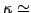0 or 1, the first four lines initialize the array currentState[k] with numberOfRealisations samples of one single price stored in a one dimensional array with an idle index ; the entire array is (arbitrarily or, rather, for plotting) initialized with the strike price currentState[k]=strike. The value of the selector scheme decides if the modeling of an in-/out-barrier option with-/out particle plotting requires the creation of an additional marker array mark[k], which has to be initialized with the corresponding behavior. Not shown in the code above but visible in the VMARKET listing, is that a parameter kappa sufficiently different from zero or one can be used to initialize a two dimensional array currentState[k][j] with j=0,..., mesh.size()-1 different prices; these are evolved in parallel if the problem is not separable-e.g. when the increments depend in a non-trivial manner on the asset price.
Starting from an initial value, possible future realizations of the asset prices are calculated with a sequence of small steps in time using (4.5.1#eq.1); in the case of a log-normal random walk (), new values for currentState[k] are calculated in MCSSolution.java repeating for each step
double timeStep = runData.getParamValue("TimeStep");
double strike   = runData.getParamValue("StrikePrice");
double mu       = runData.getParamValue("Drift");
double divid    = runData.getParamValue("Dividend");
double sigma    = runData.getParamValue("Volatility");
double barrier  = runData.getParamValue("Barrier");

if(Math.abs(kappa-1.)<0.001){               //Separable log-normal
for(int k=0; k<numberOfRealisations; k++)
currentState[k]+= currentState[k]*( (mu-divid)*timeStep +
random.nextGaussian()*sigma*Math.sqrt(timeStep) );

//Barriers
if (scheme.equals(vmarket.MCOUT) || scheme.equals(vmarket.MCOUTPP)){
for(int k=0; k<numberOfRealisations; k++)
if ((barrier >= 0. && currentState[k]-strike > strike*barrier)||
(barrier <  0. && currentState[k]-strike < strike*barrier)  )
mark[k]=0.;
} else if (scheme.equals(vmarket.MCIN) || scheme.equals(vmarket.MCINPP)){
for(int k=0; k<numberOfRealisations; k++)
if ((barrier >= 0. && currentState[k]-strike > strike*barrier)||
(barrier <  0. && currentState[k]-strike < strike*barrier)  )
mark[k]=1.;
}
}
The first four lines compute the deterministic (mu-divid)*timeStep and the random component random.nextGaussian()*sigma*Math.sqrt(timeStep) of the evolution, which are easily identified as the right-hand side of (4.5.1#eq.1). Further scaling by the underlying asset price currentState[k] reproduces the log-normal distribution of the increments, which are finally accumulated with the Java operator currentState[k]+=increment. The variable mark[k] is reset to zero (alt. one) whenever the condition for an out-'' (alt. in-'') barrier is met for a given sample. Note that the position of the barrier is here defined relative to the initial condition, using a positive (alt. negative) value of the variable barrier to distinguish a barrier above (alt. below) the initial price of the underlying. This relative definition is here required to keep the problem separable, so that the evolution of any price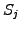can be obtained from the same sequence of increments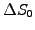normalized to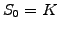using the scaling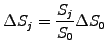(4.5.1#eq.2)

These values need in fact not to be evaluated until the expected values of the derivatives are calculated from the underlying in a manner described in the comming section.
Using a finite time step in (4.5.1#eq.1) is of course an approximation of the stochastic differential (3.3.1#eq.1); great care has to be taken to keep the steps small enough not to create, for example, negative asset prices when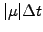or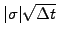exceed unity in a log-normal walk. For the record, note that the stochastic differential (assuming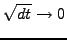) is not an exact model neither, since a finite number of trades sets a lower limit on the duration between trades on the markets. The VMARKET applet below illustrates some effects of the time discretization for the case of an down-and-in barrier put option.

";

 Virtual market experiments: Monte-Carlo random walk Increase the value of the TimeStep parameters to create spurious negative asset values with a bad numerical approximation of a log-normal random walk. Choose the largest TimeStep that you believe gives a financially significant result; justify your choice. Switch to a normal evolution by setting LogNkappa=0 and discuss what is now the upper limit for the time step.

Having dealt with the simulation of the underlying asset price, we now turn to the valuation of derivatives.

SYLLABUS  Previous: 4.5 Methods for European  Up: 4.5 Methods for European  Next: 4.5.2 Expected value of